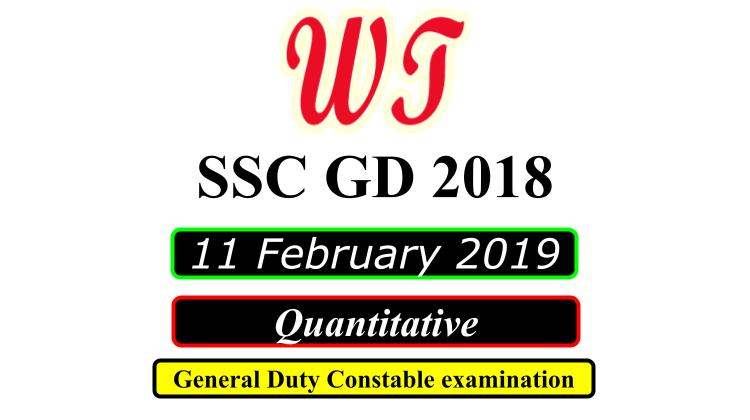Type Here to Get Search Results !

Download SSC (Staff Selection Commission) GD (General Duty), 11 February 2019 ( Math )Quantitative Questions PDF With Answers.

Table of Content

Table of Content (toc)SSC GD 11 February 2019 Quantitative Questions PDF Download Free

### SSC GD 11 Feb 2019 Shift 1 Math Questions

Q3:-Rajesh goes to his office at the speed of 25 km/hr and returns to his home at the speed of 20 km/hr. If he takes total 9 hours, then what is the distance between his office and home?
A.    120km
B.    90km
C.    140km
D.    100km
Q4:-A sum becomes ₹ 10650 in 5 years and ₹ 11076 in 6 years at simple interest. What is the sum?
A.    ₹ 8946
B.    ₹ 8740
C.    ₹ 8520
D.    ₹ 8800
Q5:-If cost price of an article is ₹220 and profit is 20%, then what will be the selling price of the article?
A.    ₹264
B.    ₹216
C.    ₹176
D.    ₹256
Q6:-The height of a cone is 16 cm and radius of its base is 30 cm. If the rate of painting it is ₹14/cm , then what will be the total cost in painting the curved surface of cone from outside?
A.    ₹42220
B.    ₹44880
C.    ₹36820
D.    ₹46540
Q7:-A sum becomes 5 times of itself in 3 years at compound interest (interest is compounded annually). In how many years will the sum becomes 125 times of itself?
A.    9
B.    6
C.    8
D.    12

### SSC GD 11 Feb 2019 Shift 2 Math Questions

Instructions
For the following questions answer them individually
Q1:-A person purchased 12 bags of sugar at the rate of ₹ 1200 per bag, 8 bags at the rate of ₹ 1500 per bag and 10 bags at the rate of ₹2100 per bags. What will be the average cost of all the bags together?
A.    ₹ 1420
B.    ₹ 1680
C.    ₹ 1580
D.    ₹ 1640
Q3:-A bowler has taken 0, 3, 2, 1, 5, 3, 4, 5, 5, 2, 2, 0, 0, 1 and 2 wickets in 15 consecutive matches. What is the median of the given data?
A.    1
B.    3
C.    0
D.    2
Q4:-A sum of ₹ 9800 gives simple interest of ₹ 4704 in 6 years. What will be the rate of interest per annum?
A.    9%
B.    8.5%
C.    8%
D.    7.5%
Q6:-If Rohit can cover a distance of 1188 km in 22 hours, then what is the speed of Rohit?
A.    54 m/s
B.    25 m/s
C.    15 m/s
D.    48 m/s
Q7:-The average weight of a group of 24 students increases by 2 kg when the weight of teacher is also included. If the initial average weight of the group of 24 students was 30 kg, then what is the weight of teacher?
A.    32 kg
B.    82 kg
C.    80 kg
D.    92 kg

### SSC GD 11 Feb  2019 Shift 3 Math Questions

Q2:-If Manoj purchases 324 toffees for ₹ 486, then how many toffees should he sell for ₹ 90 to earn 50% profit?
A.    40
B.    60
C.    55
D.    45
Q4:-Ravi, Manish and Naveen alone can complete a work in 30 days, 15 days and 10 days respectively. They start the work together but Ravi leaves the work after 2 days of the starting of the work and Manish leaves the work after 3 days more. In how many days Naveen will complete the remaining work?
A.    3 days
B.    4 days
C.    1 day
D.    2 days
Q5:-A sum of ₹ 10640 gives interest of ₹ 3724 in x years at 5% simple interest. What will be the value of x ?
A.    8 years
B.    6 years
C.    7 years
D.    9 years
Q6:-The Least Common Multiple and Highest Common Factor of two numbers are 60 and 4 respectively. If their sum is 32, then what will be the difference of these two numbers?
A.    4
B.    8
C.    12
D.    20
Q7:-After giving a discount of 20%, a shopkeeper earns 36% profit. Marked price is what percent more than the cost price?
A.    60%
B.    76%
C.    68%
D.    70%

SSC GD 2018

SSC GD Previous Year Paper

SSC GD Previous Year English Questions

SSC GD Previous Year Reasoning Questions

SSC GD Previous Year Quantitative Questions